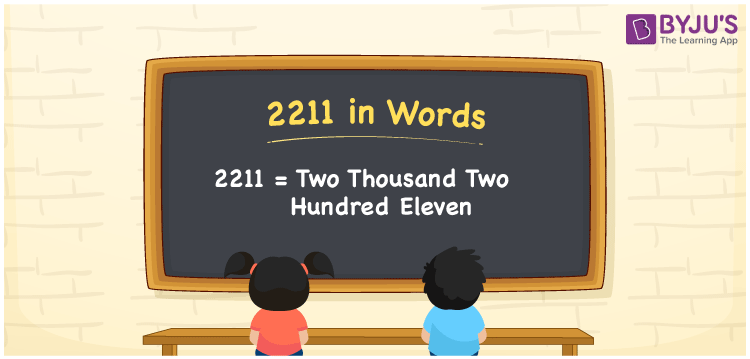# 2211 in Words

The number 2211 in words is written as “two thousand two hundred eleven”. If you bought a cycle for Rs. 2211, it can be written as “I bought a cycle for Rs. Two thousand two hundred eleven. Also, the number 2211 is a cardinal number, as it specifies the particular quantity.

 2211 in Words: Two Thousand Two Hundred Eleven. Two Thousand Two Hundred Eleven in Numerical Form: 2211.

## 2211 in English Words## How to Write 2211 in Words?

The place values of 2211 are given below up to four digits.

 Thousands Hundreds Tens Ones 2 2 1 1

The expanded form of 2211 is as follows:

= 2 × Thousand + 2 × Hundred + 1 × Ten + 1 × One

= 2 × 1000 + 2 × 100 + 1 × 10 + 1 × 1

= 2000 + 200 + 10 + 1

= 2211

= Two thousand two hundred eleven

Hence, 2211 in words is two thousand two hundred eleven.

2211 in words – Two thousand two hundred eleven

Is 2211 an odd number? – Yes

Is 2211 an even number? – No

Is 2211 a perfect square number? – No

Is 2211 a perfect cube number? – No

Is 2211 a prime number? – No

Is 2211 a composite number? – Yes

## Frequently Asked Questions on 2211 in Words

Q1

### How to spell 2211 in words?

2211 in words is two thousand two hundred eleven.

Q2

### Simplify 2200 + 11, and express it in words.

Simplifying 2200 + 11, we get 2211. Hence, 2211 in words is two thousand two hundred eleven.

Q3

### Is 2211 an odd number?

Yes, 2211 is an odd number.